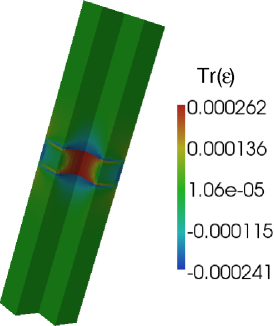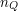## Selfconsistent EFA-Drift-diffusion 3D calculation for a GaAs quantum well in a nanocolumn

In this application, we show the results of fully Self-Consistent Schroedinger (EFA) - Drift-diffusion calculations for a 3D nanostructure: an AlGaAs rectangular nanocolumn p-i-n diode structure with an embedded GaAs quantum well.

Here is an overview of the approach chosen for self-consistent calculation in tiberCAD.

First, the solution of the eigenvalue problems resulting from the quantum EFA model provides the energy spectrum and the particle densities. The particle densities are calculated by populating the electron and hole states according to the expectation value of the corresponding electrochemical potential; then they are fed back to the Poisson/drift-diffusion model for self-consistent Schroedinger–Poisson/drift-diffusion calculations. These can be classified as overlap type simulations, where the results of the nanoscale calculation acts as an input – e.g. in form of parameters – to the microscale simulation.

## Device structure

This is the structure of the 170 nm-high nanocolumn p-i-n diode: a 4 nm-thick GaAs intrinsic active region (quantum well), the Al0.3GaAs barriers (4 nm each) and two highly doped Al0.3GaAs contact regions (respectively p-type and n-type). The section of the nanocolumn is 9 nm-wide.## 1. Strain and Drift-diffusion

### top

First of all we perform the calculations of strain and drift-diffusion transport
In fact, first we want to use strain results for the drift-diffusion current calculation. Then, the information about band structure and Fermi level position is used in the quantum calculation to calculate the correct occupation of quantum states.
We choose a bias of 1.75 V to get a reasonable population of the states in the quantum well.

Here  is the  trace of the strain tensor,  corresponding to the relative volumic change. As AlGaAs and GaAs have very similar lattice constants, the strain in this particular system is quite small and therefore of minor importance.
Also, no piezoelectric or spontaneous polarization is present here, for a (001)-grown GaAs structure.## 2. Quantized states of electrons and holes

### top

Self-consistent calculations make use of the quantized states of electrons and holes in the quantum well (QW). Thus, we solve Schrödinger equation for electrons and holes in the quantum region which comprises the GaAs QW and two AlGaAs barrier regions in the nanocolumn at the sides of the QW.

In particular we specify:

• 10 and 32 eigenstates to be calculated respectively for electrons and holes
• electric potential and strain are applied
• a 6x6 k.p model for holes and a single band (conduction band) model for electrons
• calculation of quantum charge density for electrons and holes

## 3. Self consistent Schroedinger-Poisson-Drift-Diffusion

### top

We choose to calculate charge density with a self-consistent calculation: drift-diffusion and quantum   simulations are solved self-consistently until convergence is obtained. The selfconsistent loop is implemented using a simple predictor-corrector scheme, which assumes that the quantum densityvaries with the potentials as the classical density.

Under this assumption the predictor for the electron density to enter the
drift-diffusion model during theselfconsistent iteration readswhereis the potential after theiteration, andis the correction to the
potential that has to be calculated.

As the electron and hole states in the system are calculated applying closed boundary conditions, the quantum densities near the interface between quantum mechanical and classical simulation domains suffer from artifical behaviour. To obtain a continuous transition from purely classical to purely quantum mechanical densities we define an embracing region with an extension of a few nanometers (here just 1 nm), where we use a linear combination of the two.

Both electron and hole densities are calculated in the quantum model and fed back to Poisson-Drift-diffusion model.

At the end of the self-consistent loop, we get the self-consistent solutions for the quantities obtained from the simulations belonging to the self-consistent loop.

Below, the band profiles and electrochemical potentials along the z-axis for selfconsistent and classical solutions are  compared. A flattening of the electrochemical potentials can be observed. This is due to the fact that the quantum mechanical carrier densities spread into the AlGaAs barriers, leading there to a higher density compared with the classical case. Therefore, a lower gradient of the electrochemical potential is needed to maintain a nearly constant particle flux across the barrier.Here are the selfconsistent and classical particle densities along the same growing direction (z-axis)Electron(left) and hole (right) density distribution in the intrinsic (active) region of the column.
A good confinement to the center of the well is observed for both electrons  and holes.